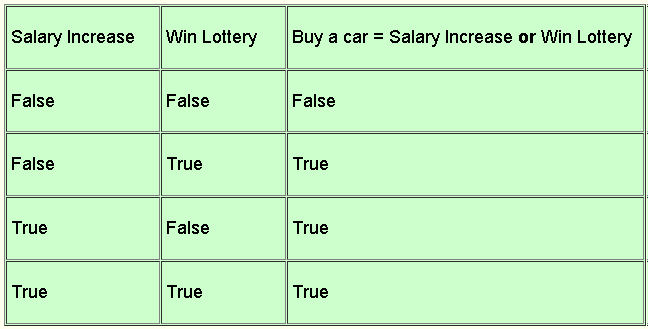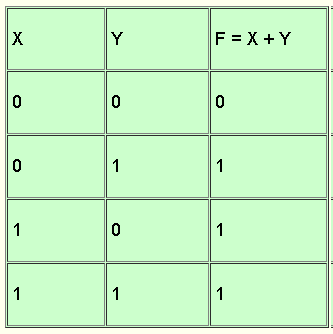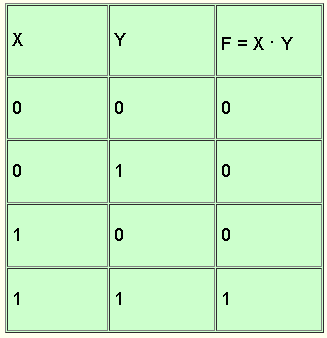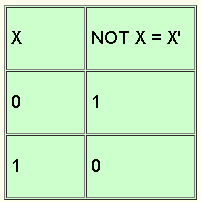# Boolean Algebra

## Introduction to Boolean Algebra

Boolean algebra which deals with two-valued (true / false or 1 and 0) variables and functions find its use in modern digital computers since they too use two-level systems called binary systems.
Let us examine the following statement:"I will buy a car If I get a salary increase or I win the lottery." This statement explains the fact that the proposition "buy a car" depends on two other propositions "get a salary increase" and "win the lottery". Any of these propositions can be either true or false hence the table of all possible situations:The mathematician George Boole, hence the name Boolean algebra, used 1 for true, 0 for false and + for the or connective to write simpler tables. Let X = "get a salary increase", Y = "win the lottery" and F = "buy a car". The above table can be written in much simpler form as shown below and it defines the OR function.Let us now examine the following statement:"I will be able to read e-books online if I buy a computer and get an internet connection." The proposition "read e-books" depends on two other propositions "buy a computer" and "get an internet connection". Again using 1 for True, 0 for False, F = "read e-books" , X = "buy a computer", Y = "get an internet connection" and use . for the connective and, we can write all possible situations using Boolean algebra as shown below. The above table can be written in much simpler form as shown below and it defines the AND function.We have so far defined two operators: OR written as + and AND written . . The third operator in Boolean algebra is the NOT operator which inverts the input. Whose table is given below where NOT X is written as X'.The 3 operators are the basic operators used in Boolean algebra and from which more complicated Boolean expressions may be written. Example: F = X . (Y + Z)

## Rules and Theorems in Boolean Algebra

X + X = X
X . X = X
(X')' = X
X + X' = 1
X . X' = 0
X + Y = Y + X , X . Y = Y . X : commutative
X + (Y + Z) = (X + Y) + Z , X . (Y . Z) = (X . Y) . Z : associative
X . (Y + Z) = X . Y + X . Z
DeMorgan's Theorem
(x + Y)' = X' . Y'
(x . Y) = X' + Y'

## References and Books

• Mendelson, E., "Introduction to Boolean Algebra and Switching Circuits". New York: McGraw-Hill, 1973.
• Steven Givant and Paul Halmos, "Introduction to Boolean Algebras (Undergraduate Texts in Mathematics)", Springer, ISBN-13: 978-0387402932.
• J. Eldon Whitesitt, "Boolean Algebra and Its Applications" (Dover Books on Mathematics), ISBN-13: 978-0486684833.